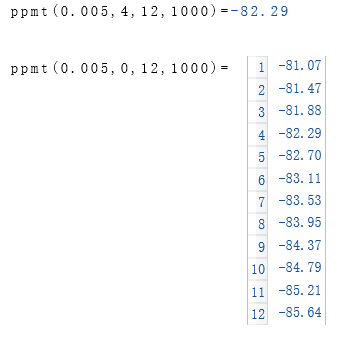# Online Calculator - PPmt Function

Returns the principal payment for a given period

## Description of the function PPmt

The PPmt funkcion returns a value specifying the principal payment for a given period of an annuity based on periodic fixed payments and a fixed interest rate.

An annuity is a series of fixed cash payments made over a period of time. An annuity can be a loan (such as a home mortgage) or an investment (such as a monthly savings plan).

The Rate and NPer arguments must be calculated using payment periods expressed in the same units. For example, if Rate is calculated using months, NPer must also be calculated using months.

For all arguments, cash paid (such as deposits to savings) is represented by negative numbers; cash received (such as dividend checks) is represented by positive numbers.

## Calculate the payment for a given period online

Rate
Interest rate
Per
Payment period
NPer
Number of periods
PV
Present value
FV
Future value
Due

Decimal places

### Result

Principal payment

## Description of the parameters

Rate

The interest rate per period. For example, if you get a car loan at an annual percentage rate (APR) of 10 percent and make monthly payments, the rate per period is 0.1/12, or 0.0083.

Per

The payment period in the range 1 through NPer.

NPer

The total number of payment periods in the annuity. For example, if you make monthly payments on a four-year car loan, your loan has a total of 4 x 12 (or 48) payment periods.

PV

The current value of a series of future payments or receipts. For example, when you borrow money to buy a car, the loan amount is the present value to the lender of the monthly car payments you will make.

FV

The future value or cash balance you want after you have made the final payment. For example, the future value of a loan is $0 because that is its value after the final payment. However, if you want to save$50,000 over 18 years for your child's education, then \$50,000 is the future value. The default ist 0.

Due

Due date specifies when payments are due. This argument must be either End of Period if payments are due at the end of the payment period, or Begin of Period if payments are due at the beginning of the period.

## RedCrab Calculator

With the "RedCrab Calculator" use the "PPmt" function for the calculation of the payment for a given period or a list of all periods.

### Example